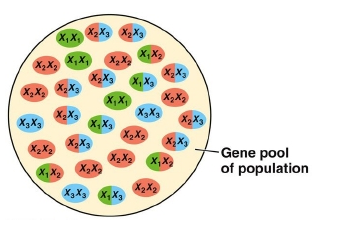# Problem: Refer to the figure below. Which description of the gene pool shown is correct?a. Size of gene pool = 30b. Frequency of X1= 0.80c. Population size = 60d. Frequency of X1X3 = 0.10e. Number of genes = 2

###### Problem Details

Refer to the figure below. Which description of the gene pool shown is correct?a. Size of gene pool = 30

b. Frequency of X1= 0.80

c. Population size = 60

d. Frequency of X1X3 = 0.10

e. Number of genes = 2

What scientific concept do you need to know in order to solve this problem?

Our tutors have indicated that to solve this problem you will need to apply the Genetic Variation concept. You can view video lessons to learn Genetic Variation. Or if you need more Genetic Variation practice, you can also practice Genetic Variation practice problems.

What is the difficulty of this problem?

Our tutors rated the difficulty ofRefer to the figure below. Which description of the gene poo...as high difficulty.

How long does this problem take to solve?

Our expert Biology tutor, Kaitlyn took 9 minutes and 38 seconds to solve this problem. You can follow their steps in the video explanation above.

What professor is this problem relevant for?

Based on our data, we think this problem is relevant for Professor Bond's class at UF.